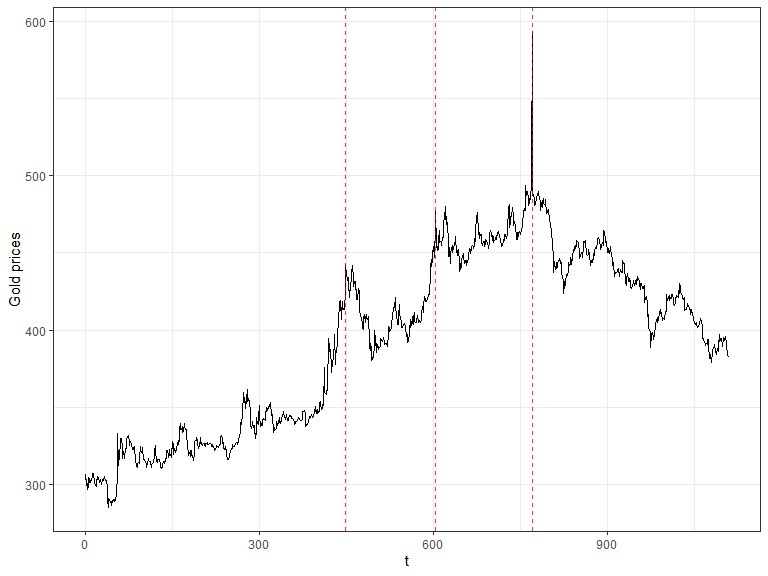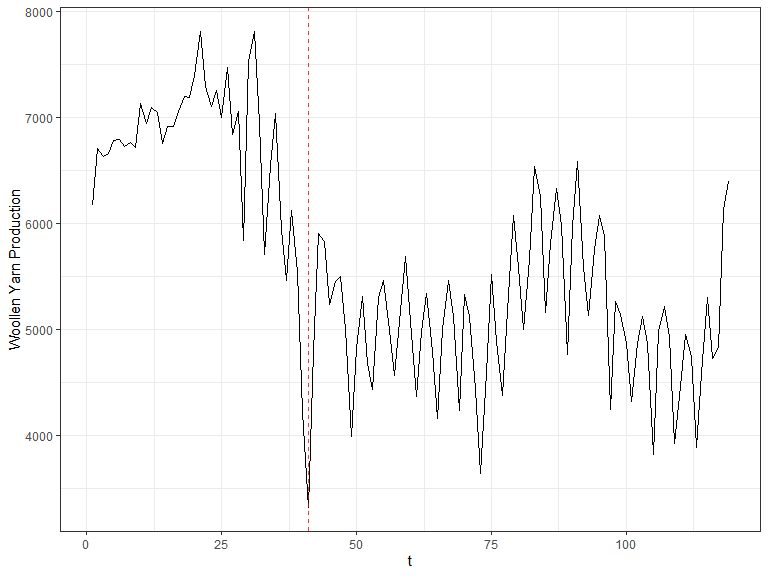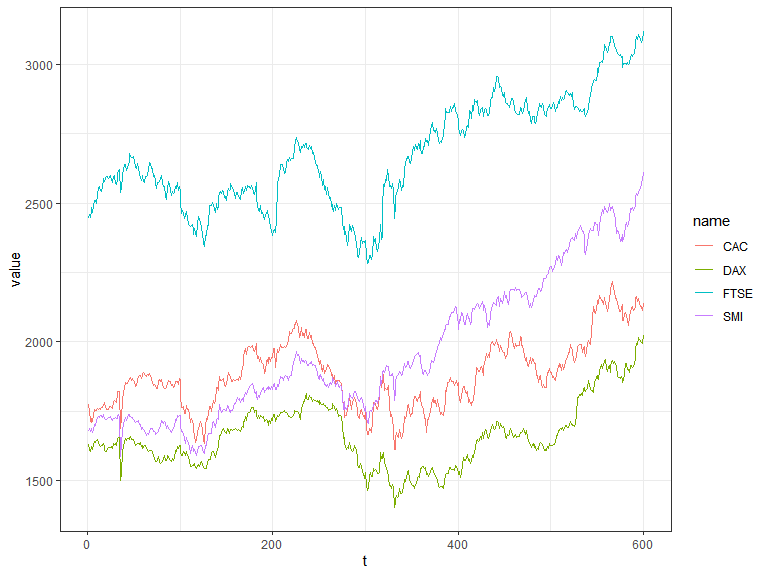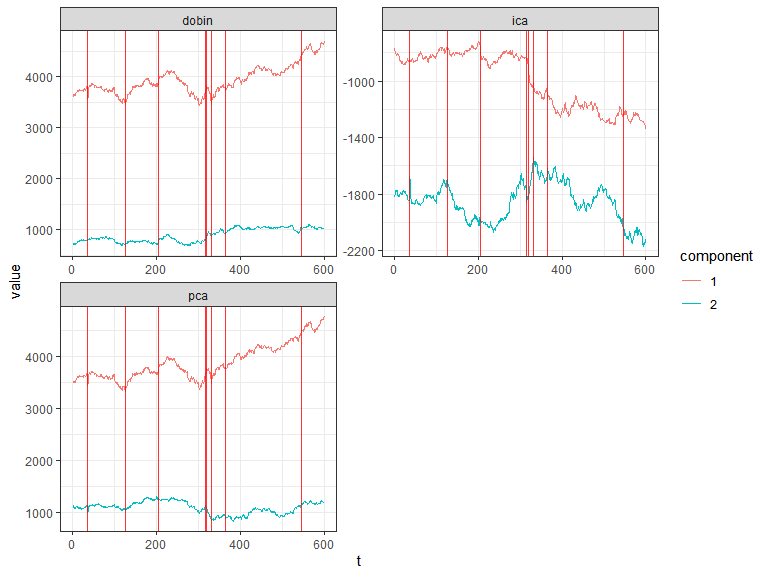# Introduction to composits

``````library(composits)
library(ggplot2)
#> Warning: package 'ggplot2' was built under R version 3.6.3
library(forecast)
library(dplyr)
#>
#> Attaching package: 'dplyr'
#> The following objects are masked from 'package:stats':
#>
#>     filter, lag
#> The following objects are masked from 'package:base':
#>
#>     intersect, setdiff, setequal, union
library(tidyr)
#> Warning: package 'tidyr' was built under R version 3.6.3
library(stringr)
library(broom)
#> Warning: package 'broom' was built under R version 3.6.3
library(rgdal)
#> Warning: package 'rgdal' was built under R version 3.6.3
#> Warning: package 'sp' was built under R version 3.6.3
#> rgdal: version: 1.5-8, (SVN revision 990)
#> Geospatial Data Abstraction Library extensions to R successfully loaded
#> Loaded GDAL runtime: GDAL 3.0.4, released 2020/01/28
#> Path to GDAL shared files: C:/Users/Sevvandi/Documents/R/win-library/3.6/rgdal/gdal
#> GDAL binary built with GEOS: TRUE
#> Loaded PROJ runtime: Rel. 6.3.1, February 10th, 2020, [PJ_VERSION: 631]
#> Path to PROJ shared files: C:/Users/Sevvandi/Documents/R/win-library/3.6/rgdal/proj
#> To mute warnings of possible GDAL/OSR exportToProj4() degradation,

The goal of composits is to find outliers in compositional, multivariate and univariate time series. It is an outlier ensemble method that uses the outlier detection methods from R packages `forecast`, `tsoutliers`, `anomalize` and `otsad`. All the options provided in those packages can be included in the calls for each of the methods used in the ensemble function so that the user can create a more customize ensemble.

As describe in the paper four dimension reduction methods are used in the multivariate ensemble PCA, DOBIN, ICS and ICA. It is recomended that users verified the scaling and centering options of these methods for each particular example.

## Univariate time series outliers

This example uses a univariate time series containing the daily gold prices from the R package `forecast`.

``````gold2 <- forecast::na.interp(gold)
out <- uv_tsout_ens(gold2)
#> Converting from tbl_df to tbl_time.
#> Auto-index message: index = date
#> frequency = 7 days
#> trend = 91 days
inds <- names(which(table(out\$outliers) > 2))

ts_gold <- dplyr::as_tibble(gold2) %>% mutate(t = 1:length(gold2)) %>% rename(value = x)
ggplot(ts_gold, aes(x=t, y=value)) +
geom_line() +
geom_vline(xintercept =as.numeric(inds), color="red", alpha=0.8, size=0.5, linetype ='dashed') + ylab("Gold prices") +
theme_bw()
#> Don't know how to automatically pick scale for object of type ts. Defaulting to continuous.``````The red dashed vertical lines show the time points that have been picked by 3 or more outlier detection methods.

Next we look at the quarterly production of woollen yarn in Australia. This time series is also taken from the R package `forecast`.

``````out <- uv_tsout_ens(woolyrnq)
#> Converting from tbl_df to tbl_time.
#> Auto-index message: index = date
#> frequency = 7 days
#> trend = 59.5 days
inds <- names(which(table(out\$outliers) > 2))

ts_wool <- dplyr::as_tibble(woolyrnq) %>% mutate(t = 1:length(woolyrnq)) %>% rename(value = x)
ggplot(ts_wool, aes(x=t, y=value)) +
geom_line() +
geom_vline(xintercept =as.numeric(inds), color="red", alpha=0.8, size=0.5, linetype ='dashed') + ylab("Woollen Yarn Production") +
theme_bw()
#> Don't know how to automatically pick scale for object of type ts. Defaulting to continuous.``````## Multivariate time series outliers

This example includes EU stock market data from the package datasets. It contains the daily closing prices of 4 European stock indices: Germany DAX, Switzerland SMI, France CAC, and UK FTSE. First we plot the data.

``````stpart <- EuStockMarkets[1:600, ]
stpart <- EuStockMarkets[1:600, ]
as_tibble(stpart) %>% mutate(t = 1:n())   %>%
pivot_longer(cols=1:4)  %>%
ggplot2::ggplot( ggplot2::aes(x = t, y = value, color = name)) +  ggplot2::geom_line() +  ggplot2::theme_bw() ``````Then we find multivariate outliers. To find the multivariate outliers, first we decompose the time series to univariate outliers by using Principle Component Analysis (PCA), Independent Component Analysis (ICA), DOBIN (Distance based Outlier BasIs using Neighbours) and ICS (Invariant Coordinate Selection) decomposition methods. The `fast=TRUE` option leaves out ICS decomposition. Then for a selected number of components (default being 2) we find outliers using the univariate time series ensemble. The outliers are given in the table below.

``````out <- mv_tsout_ens(stpart, fast=TRUE)
#> Converting from tbl_df to tbl_time.
#> Auto-index message: index = date
#> frequency = 7 days
#> trend = 91 days
#> Converting from tbl_df to tbl_time.
#> Auto-index message: index = date
#> frequency = 7 days
#> trend = 91 days
#> Converting from tbl_df to tbl_time.
#> Auto-index message: index = date
#> frequency = 7 days
#> trend = 91 days
#> Converting from tbl_df to tbl_time.
#> Auto-index message: index = date
#> frequency = 7 days
#> trend = 91 days
#> Converting from tbl_df to tbl_time.
#> Auto-index message: index = date
#> frequency = 7 days
#> trend = 91 days
#> Converting from tbl_df to tbl_time.
#> Auto-index message: index = date
#> frequency = 7 days
#> trend = 91 days
#> Converting from tbl_df to tbl_time.
#> Auto-index message: index = date
#> frequency = 7 days
#> trend = 91 days
#> Converting from tbl_df to tbl_time.
#> Auto-index message: index = date
#> frequency = 7 days
#> trend = 91 days
#> Converting from tbl_df to tbl_time.
#> Auto-index message: index = date
#> frequency = 7 days
#> trend = 91 days
#> Converting from tbl_df to tbl_time.
#> Auto-index message: index = date
#> frequency = 7 days
#> trend = 91 days
#> Converting from tbl_df to tbl_time.
#> Auto-index message: index = date
#> frequency = 7 days
#> trend = 91 days
#> Converting from tbl_df to tbl_time.
#> Auto-index message: index = date
#> frequency = 7 days
#> trend = 91 days
out\$outliers
#>    Indices Total_Score Num_Coords Num_Methods     DOBIN       PCA
#> 11      36      1.6928          3           3 0.5460645 0.6552774
#> 18     127      0.3120          1           1 0.3120000 0.0000000
#> 20     205      0.8160          3           2 0.2733508 0.4059739
#> 32     316      1.4400          3           2 0.4710592 0.6156465
#> 34     319      0.6880          2           2 0.1720000 0.0000000
#> 41     331      1.5008          3           3 0.2728727 0.4093091
#> 42     366      0.3120          1           1 0.0000000 0.3120000
#> 48     546      0.3120          1           1 0.3120000 0.0000000
#>          ICA forecast tsoutliers otsad anomalize Gap_Score_2
#> 11 0.4914581        0      1.248 0.320    0.1248          72
#> 18 0.0000000        0      0.312 0.000    0.0000           5
#> 20 0.1366754        0      0.624 0.192    0.0000          30
#> 32 0.3532944        0      1.248 0.192    0.0000          60
#> 34 0.5160000        0      0.624 0.064    0.0000          23
#> 41 0.8186182        0      1.248 0.128    0.1248          63
#> 42 0.0000000        0      0.312 0.000    0.0000           5
#> 48 0.0000000        0      0.312 0.000    0.0000           5
draw_table_html(out)``````
Indices DOBIN PCA ICA Num_Coords forecast tsoutliers otsad anomalize Num_Methods Gap_Score_2 Total_Score
36 0.55 0.66 0.49 3 0 1.25 0.32 0.12 3 72 1.69
331 0.27 0.41 0.82 3 0 1.25 0.13 0.12 3 63 1.5
316 0.47 0.62 0.35 3 0 1.25 0.19 0 2 60 1.44
205 0.27 0.41 0.14 3 0 0.62 0.19 0 2 30 0.82
319 0.17 0 0.52 2 0 0.62 0.06 0 2 23 0.69
127 0.31 0 0 1 0 0.31 0 0 1 5 0.31
366 0 0.31 0 1 0 0.31 0 0 1 5 0.31
546 0.31 0 0 1 0 0.31 0 0 1 5 0.31

The decomposed time series is shown in the figure below.

``plot_decomposed_all(obj=out, X = stpart)````animate_ts_ensemble(out, X= stpart,  max_frames = 100)``